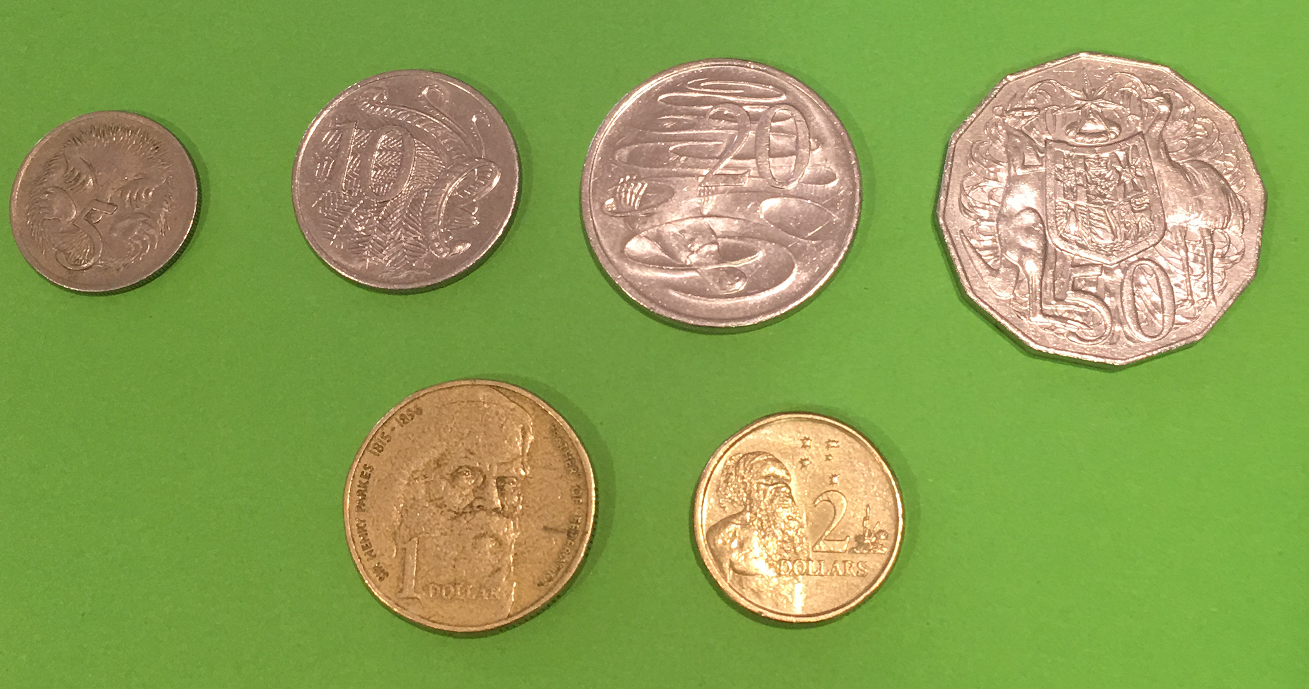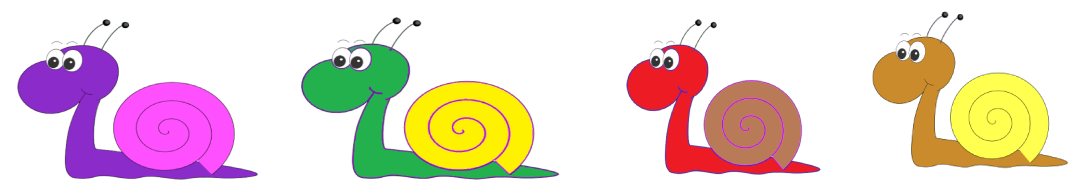# Different Types of Numbers

Cardinal numbers are counting numbers. Ordinal numbers tell us about position. Nominal numbers are just names: they don't have any value or position.

## Cardinal numbers

The cardinal numbers are the counting numbers. They are whole numbers that tell you how many of something there are. So, they don't include fractions or decimals. For example, you would use a cardinal number to describe the number of brussels sprouts that are on your plate at the start of dinner (hopefully, $0$!). In the picture below, there are six coins. Six is a cardinal number.Cardinal numbers let us count things.

## Ordinal Numbers

How do we describe the position of something in a race, a line or a list? Using an ordinal number. Ordinal numbers tell us about position. They are things like 1st (or first), 2nd(or second), 3rd (or third) 4th (or fourth), 5th (or fifth) and 6th (or sixth), etc. For example, the snails in the picture below are having a race.The pink and purple snail is winning, so he is in 1st position. The green and yellow snail is coming second, the red and brown snail is coming third and the brown and yellow snail is coming last. As there are only four snails in the race, he is in 4th position.
Ordinal numbers tell us about the order that things are in.

## Nominal Numbers

These are just numbers that are used as names. For example, the car in the picture below is given the number $453$ as a name. This name tells us nothing about how many cars there are or the position of the car in a race.Some other examples of nominal numbers include
• The number on a cricketer's shirt. Nathan Lyon wore shirt number 67 in 2016.
• A post code: Melbourne's post code is 3000.
• A lego set number such as 75192.
Nominal numbers let us name things.

### Description

This mini book covers the core of Math for Foundation, Grade 1 and Grade 2 mathematics including

1. Numbers
3. Subtraction
4. Division
5. Algebra
6. Geometry
7. Data
8. Estimation
9. Probability/Chance
10. Measurement
11. Time
12. Money
13. and much more

This material is provided free of cost for Parent looking for some tricks for their Prekinder, Kinder, Prep, Year 1 and Year 2 children

### Learning Objectives

These lessons are for kids aged 4-8 with the core objective to expose their brains to concepts of addition, subtraction, division, algebra and much more.

Author: Subject Coach
You must be logged in as Student to ask a Question.